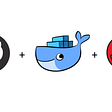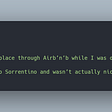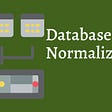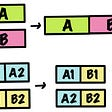# Shape Property

`import numpy as nparray = np.array([[1, 2, 3], [4, 5, 6]])print(array.shape)Output:(2, 3)`

# ndmin Argument

`import numpy as nparray = np.array([[1, 2, 3], [4, 5, 6]], ndmin=7)print(array.shape)Output:(1, 1, 1, 1, 1, 2, 3)`

# Reshape Function

`import numpy as nparray = np.array([[1, 2, 3], [4, 5, 6]])reshapedArray = array.reshape(6)print(reshapedArray)Output:[1 2 3 4 5 6]`
`import numpy as nparray = np.array([[1, 2, 3], [4, 5, 6]])reshapedArray = array.reshape(1, 3, 2)print(reshapedArray)Output:[[[1 2]  [3 4]  [5 6]]]`

# Example: Modifying Original Array After Reshaping

`import numpy as nparray = np.array([[1, 2, 3], [4, 5, 6]])reshapedArray = array.reshape(6)array = 7print(reshapedArray)Output:[7 2 3 4 5 6]`

# Example: Reshaping Error

`import numpy as nparray = np.array([[1, 2, 3], [4, 5, 6]])reshapedArray = array.reshape(3, 2, 3)print(reshapedArray)Output:ValueError: cannot reshape array of size 6 into shape (3,2,3)`

# Trick to Reshape Into a 1-D Array

`import numpy as nparray = np.array([[1, 2, 3], [4, 5, 6]])reshapedArray = array.reshape(-1)print(reshapedArray)Output:[1 2 3 4 5 6]`

# Conclusion

--

--

--

## More from Jesse L

Love podcasts or audiobooks? Learn on the go with our new app.

## Jdict (JavaScript dict) in Python## Self Storage Quarters — Garden City, NY — Addresses.com## Top 3 Programming Languages in 2020## The 3 Things I Wish I’d Known When I Began My Career in Software## Dark sides of Competitive Programming## Flutter Deep Dive, Part 3: “RenderFlex children have non-zero flex…”## Love & variables## ReLU Activation Function## Normalization## Hstack (NumPy) in Python